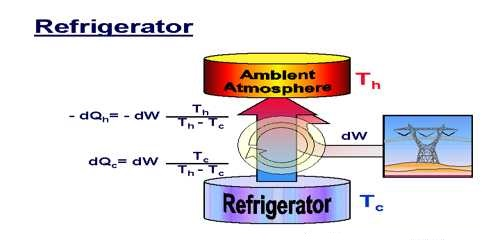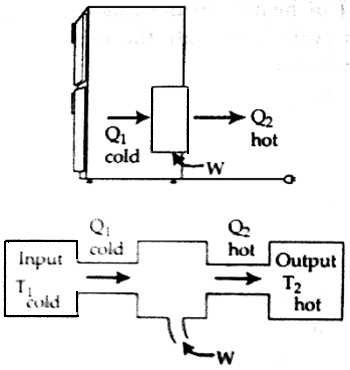Physics

# Co-efficient of Performance of a RefrigeratorCo-efficient of Performance of a refrigerator

The ratio of heat removed from the refrigerator and the mechanical work done is called co-efficient of performance. It is represented by K. Now, if Q is the heat removed by the vaporization coil [Figure], W is the work done by the compressor and Q2 is the rejected heat in the condenser coil, then according to the thermal law, we get,

Q2 = Q1 + W

W = Q2 – Q1

So according to theory co-efficient of performance,

K = [Heat removed / Supplied work] = Q1 / (Q2 – Q1) = Q1 / WThe more the co-efficient of performance, let will he the mechanical work to be done for removing more heat from the refrigerator. The value of co-efficient of performance in a refrigerator is between 2 to 6.

The efficiency of a refrigerator,

η = Q1 / W ≤ T1 / (T2 – T1)

Here, T1 = Removed temperature and T2 = Rejected temperature.

The efficiency of a refrigerator is based on the energy consumed per year for a given size. The efficiency of a refrigerator is expressed in volume cooled per unit electric energy per day. Volume is measured in cubic feet and electrical energy is measured in kilowatt hours.